### Introduction

The developments below are in the github version of `sp`, and will become part of sp release 1.2-3 and higher.

Plotting spatial grids using the `plot` method was up till now not much fun, and limited to either showing a grid, as in

``````library(sp)
plot(geometry(meuse.grid))
``````which only shows the cell geometry, or using `image` as in

``````image(meuse.grid["dist"])
``````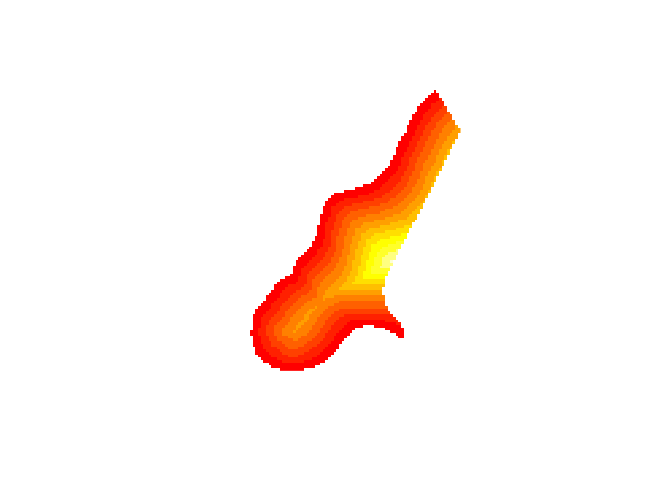For more advanced plots, showing color scales, one needed to use `spplot`. `ssplot` is a powerful function, but may also be challenging to use, plots may be challenging to fine manipulate.

The are base plots coming from `plot` can be improved by all the bells and whistles of base plot, using `par` and by incrementally adding features. The big missing thing though is a colour legend showing the z-values. Both `raster::plot` and `spatstat::plot` provide colour scales, and it is about time to add this to `sp`’s plot methods for `SpatialGridDataFrame` and `SpatialPixelsDataFrame` objecs as well. With the help of this blog entry, this wasn’t too much work.

``````plot(meuse.grid["dist"], zlim = c(0,1))
``````which shows a grid using a larger part of the plotting area, and a color scale bar. `zlim` has been set here to ensure the scale goes to 1 (the data go to 0.99).

The rest of this blog post shows the options now available to this plot method.

The new plot method uses `layout` to make two plotting areas, one for the grid and one for the scale. Since the last element plotted is the grid, we can add to it:

``````plot(meuse.grid["dist"], zlim = c(0,1))
title("distance to river Meuse (normalized)")
points(meuse, col = 'green')
box()
``````### Axes, scale placement and size

Axes can be added by specifying `axes = TRUE`, the location of the scale by specifying `axis.pos`, which follows the numbering of `?axis`. Note how the size of the scale adapts to the axes:

``````plot(meuse.grid["dist"], zlim = c(0,1), axes = TRUE)
``````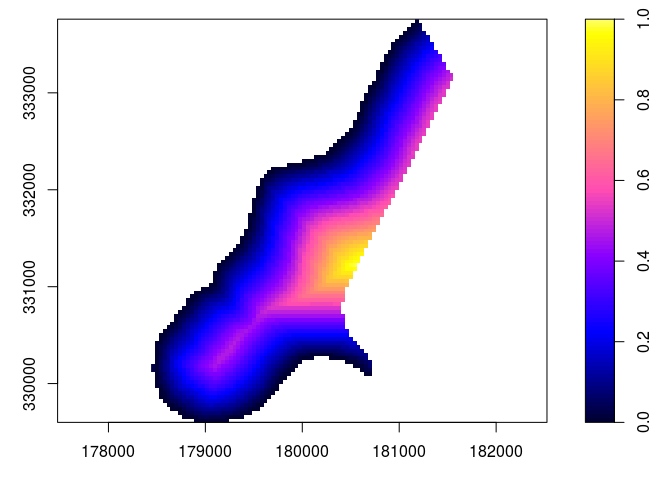``````plot(meuse.grid["dist"], zlim = c(0,1), axes = TRUE, axis.pos = 1)
``````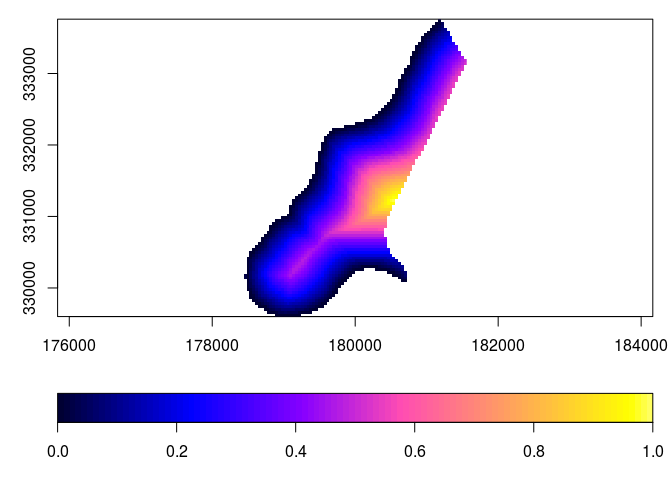The scale size can be reduced by specifying `scale.shrink`; its thickness can be modified by `scale.size`:

``````plot(meuse.grid["dist"], zlim = c(0,1), axes = TRUE, scale.shrink = 1)
``````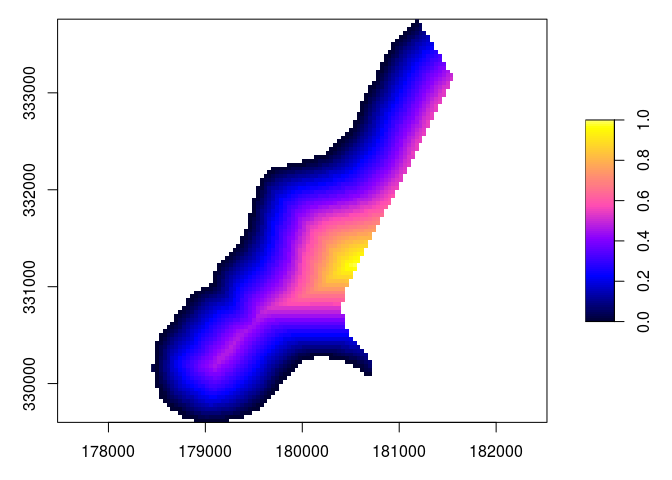``````plot(meuse.grid["dist"], zlim = c(0,1), axes = TRUE, scale.size = lcm(2.4))
``````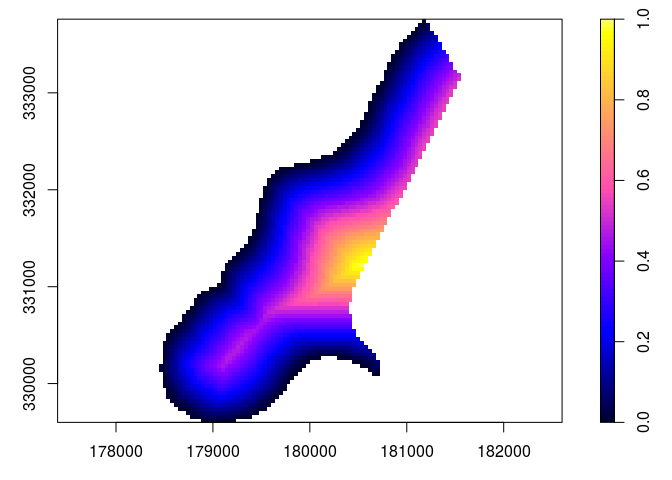where `lcm(2)` indicates 2 cm, meaning that it isn’t affected by changing plot size. If a numeric values is given here, e.g. 1/6, the size is relative to the area area occupied by the grid.

to increase the white space around the gridded area, `xaxs = "r"` can be passed, which adds the usual 4% on each side.

### Specifying the scale color breaks, tics and labels

Color breaks are specified by `breaks`, specifying `breaks` requires that a color ramp of matching length is specified (one less than the number of breaks). Tics and tic labels are defined by parameter `at`. When specifying `breaks`, `zlim` becomes obsolete.

``````b = c(0, 0.25, 0.5, 0.6, 0.7, 0.8, 0.9, 1)
col = rev(bpy.colors(length(b)-1))
plot(meuse.grid["dist"], breaks = b, col = col, at = b)
``````Grid lines can be added by specifying a grid cell `border` color, as in

``````plot(meuse.grid["dist"], border = grey(0.6))
``````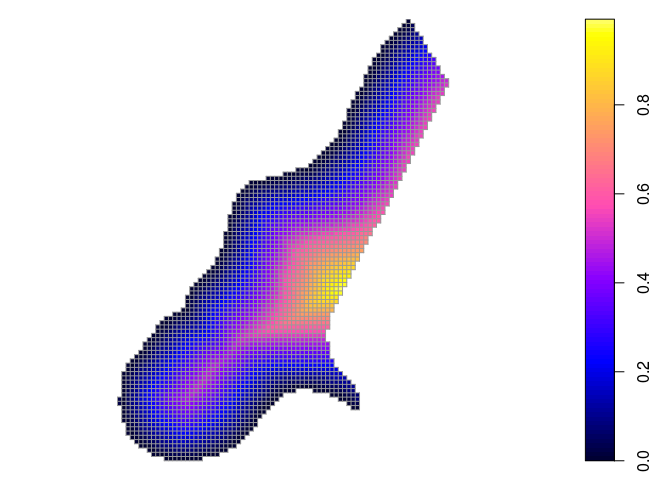This also enlightens the difference between `SpatialPixelsDataFrame` (as plotted above), and `SpatialGridDataFrame` which has the complete set of grid cells in a rectangular area:

``````library(methods)
plot(as(meuse.grid, "SpatialGridDataFrame")["dist"], border = grey(0.6))
``````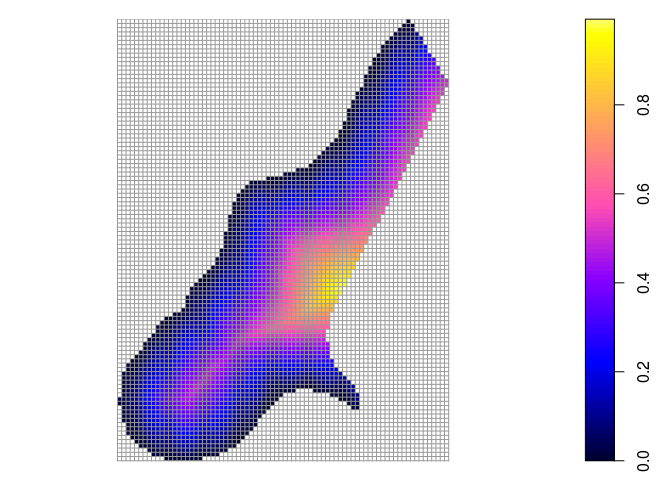### Plotting scale only, or image only

Sometimes, a user needs an image of the scale only, e.g. to glue it onto a leaflet plot when it does not properly support legends. In the following, the figure (device) width was set to 2 (inch), so that the scale still looks nice:

``````plot(meuse.grid["dist"], what = "scale", zlim = c(0,1))
``````The other option is to only plot the grid, without scale:

``````plot(meuse.grid["dist"], what = "image")
``````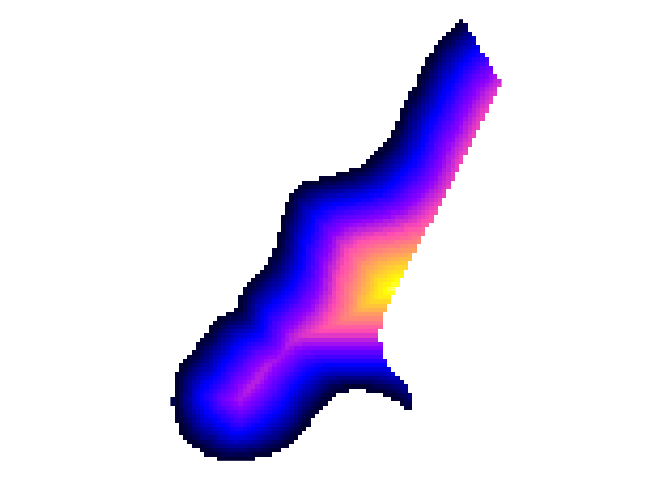### Plotting gridded categorical (factor) variables

Categorical (`factor`) variables need a different treatement, as continuous color change does not work for them, and neither do continuous color scales. The default color scale used in this case is `Set2` from the `RColorBrewer` package; see also the colorbrewer and the command `RColorBrewer::display.brewer.all()`.

``````plot(meuse.grid["ffreq"])
``````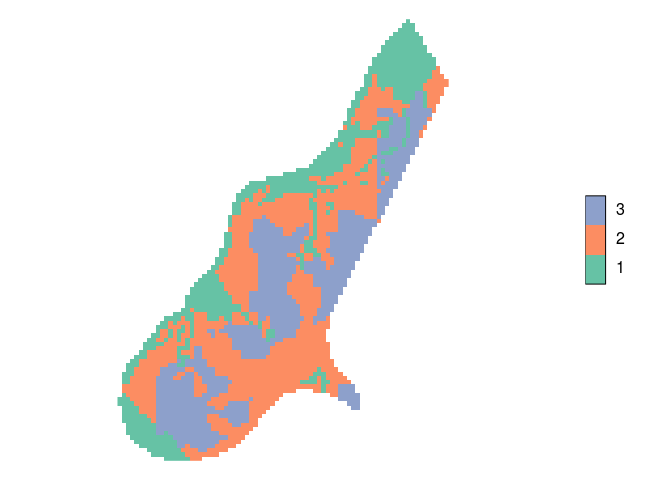``````plot(meuse.grid["ffreq"], axis.pos = 1)
``````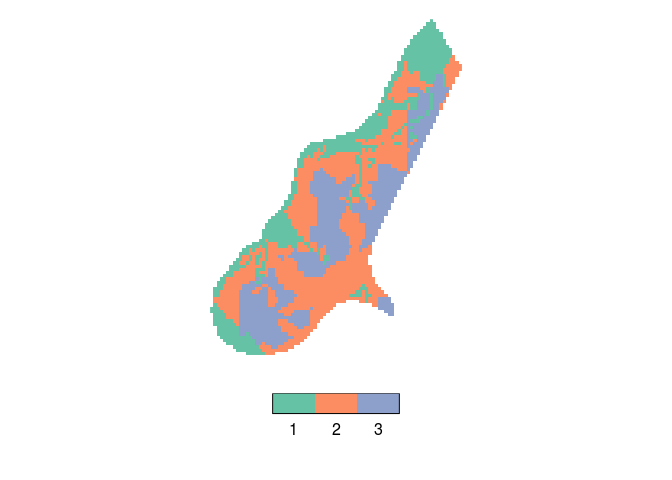### Factors: changing the density and width of scales, label width

The amount of space taken up by a colored field in a legend class can be manipulated. Argument `scale.n` determines how many fields would fill a complete side (default 15), increasing it decreases the (here vertical) size of a color field:

``````plot(meuse.grid["ffreq"], scale.n = 25)
``````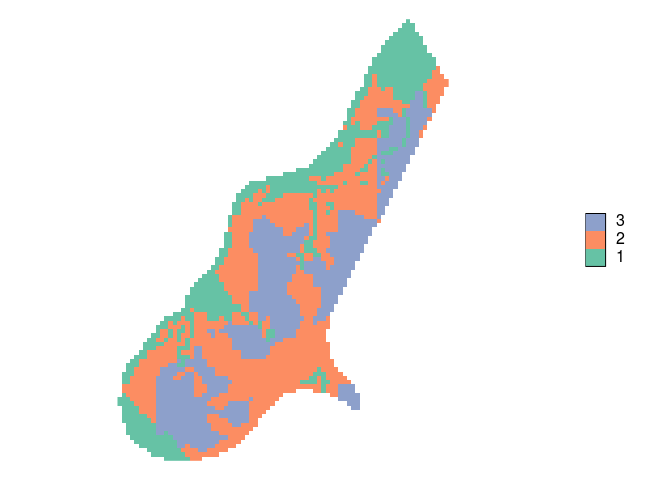Argument `scale.frac` determines which fraction of the scale area is taken by the coloured bar; in this case (`axis.pos=4`) it determines the width of the color area (default 0.3):

``````plot(meuse.grid["ffreq"], scale.frac = 0.5)
``````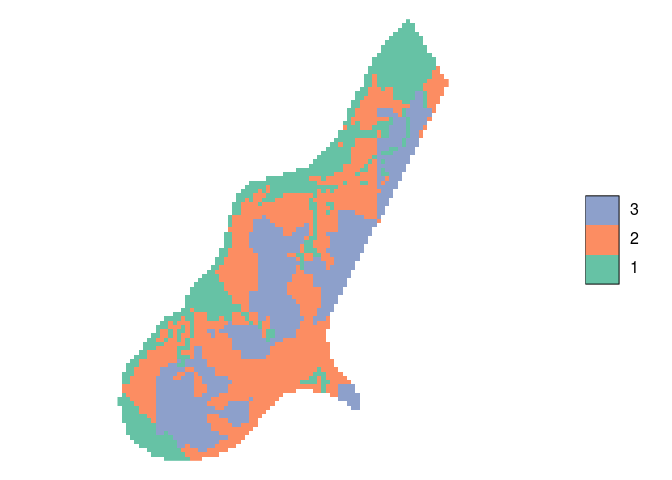In the following example, wide labels need extra space; this is created by

• increasing `scale.size`, so there is more space for the scale + labels
• decreasing `scale.frac` so that the absolute size of the bar remains the same
``````levels(meuse.grid\$ffreq) = c("frequent", "moderately frequent", "infrequent")
plot(meuse.grid["ffreq"], scale.size = lcm(5.8), scale.frac = 0.15)
``````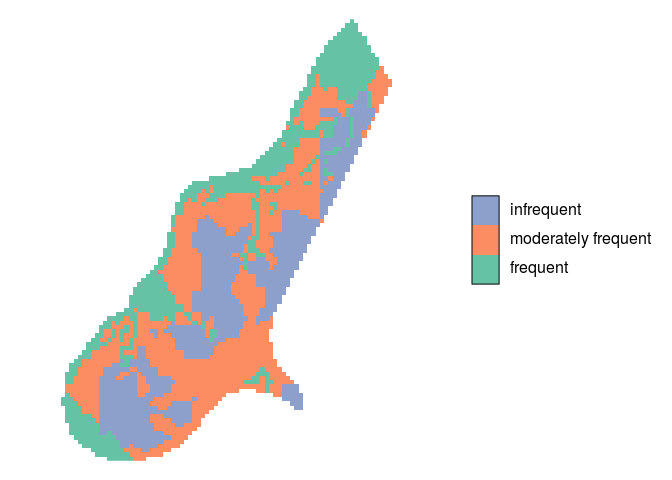### Relative size of the categorical scale

The relative size of a scale compared to the rest of the image, including its fonts, can be manipulated by changing the total size of the graph. In the following, the size is set to 12 x 12 (inch); the (automatic) resizing of the final image gives a seemingly smaller scale:

``````plot(meuse.grid["dist"])
``````### Arranging multiple plots

Multiple plots can be arranged on a single device using `layout`. Since `plot` already uses `layout` when arranging the image and the scale, simply putting two images with scales side by side is not simple – layouts cannot be nested. A solution is to create plots of only image and scale (taking care of `zlim`), and have them precede by an appropriate `layout` statement. For a shared legend, e.g. by

``````layout(matrix(1:3, 1, 3), widths = c(4,4,1))
plot(meuse.grid, what = "image", zlim = c(0,1))
plot(meuse.grid["dist"], what = "image", zlim = c(0,1))
plot(meuse.grid["dist"], what = "scale", zlim = c(0,1))
``````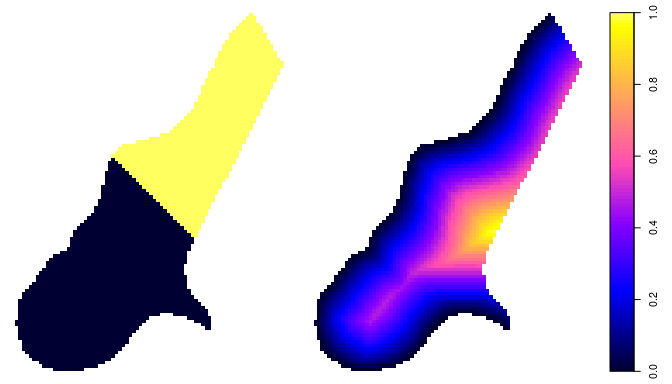and for separate legends e.g. by

``````layout(matrix(1:4, 2, 2), heights = c(4,1))
levels(meuse.grid\$ffreq) = c("fr", "mo", "inf")
plot(meuse.grid["ffreq"], what = "image")
plot(meuse.grid["ffreq"], what = "scale", axis.pos = 1)
plot(meuse.grid["dist"], what = "image", zlim = c(0,1))
plot(meuse.grid["dist"], what = "scale", axis.pos = 1, zlim = c(0,1))
``````More powerful arrangment methods are obtained when using plotting methods available in package `grid`, or higher-level plotting functions such as `spplot` or `ggplot2::ggplot`.

### Challenges

• integrate this with the plotting of other classes with attributes, in particular `SpatialPolygonsDataFrame` and `SpatialLinesDataFrame`
• get the text labels along vertical continuous scales horizontal, meaning don’t use `axis` and more managment of space
• unify the scaling of the scale bars for the two different scale types (continuous, categorical)
• deal with the multiple plot issue more elegantly